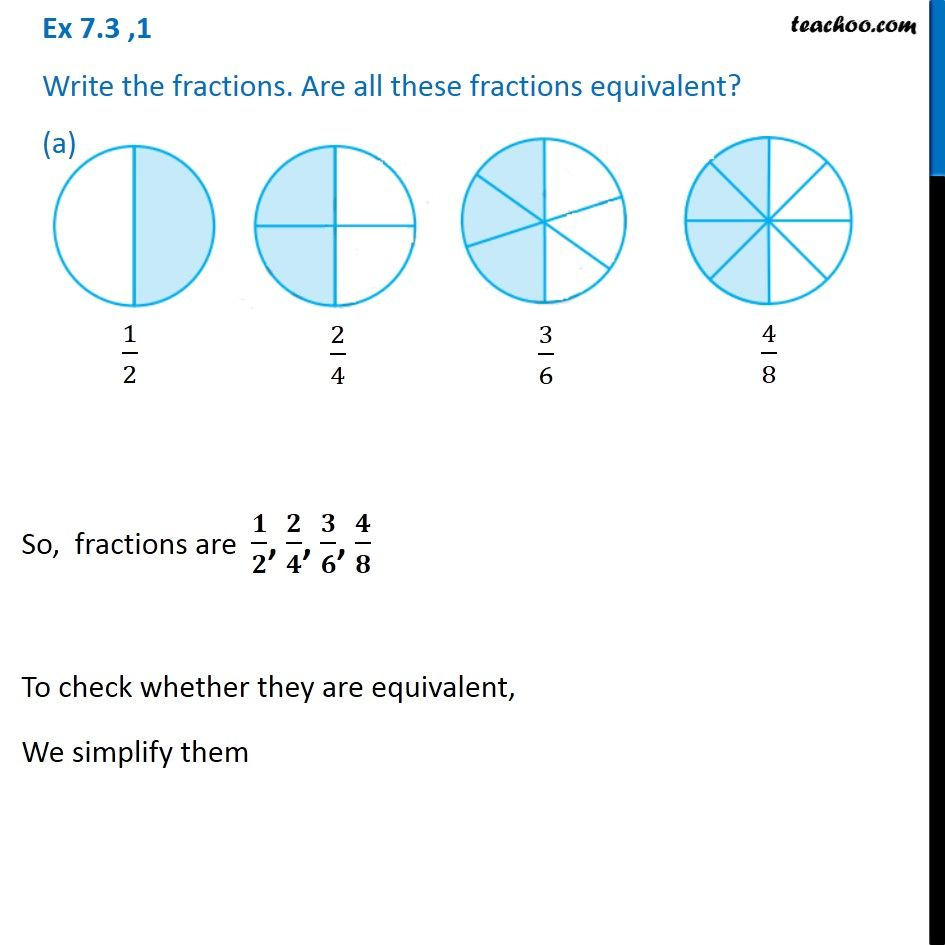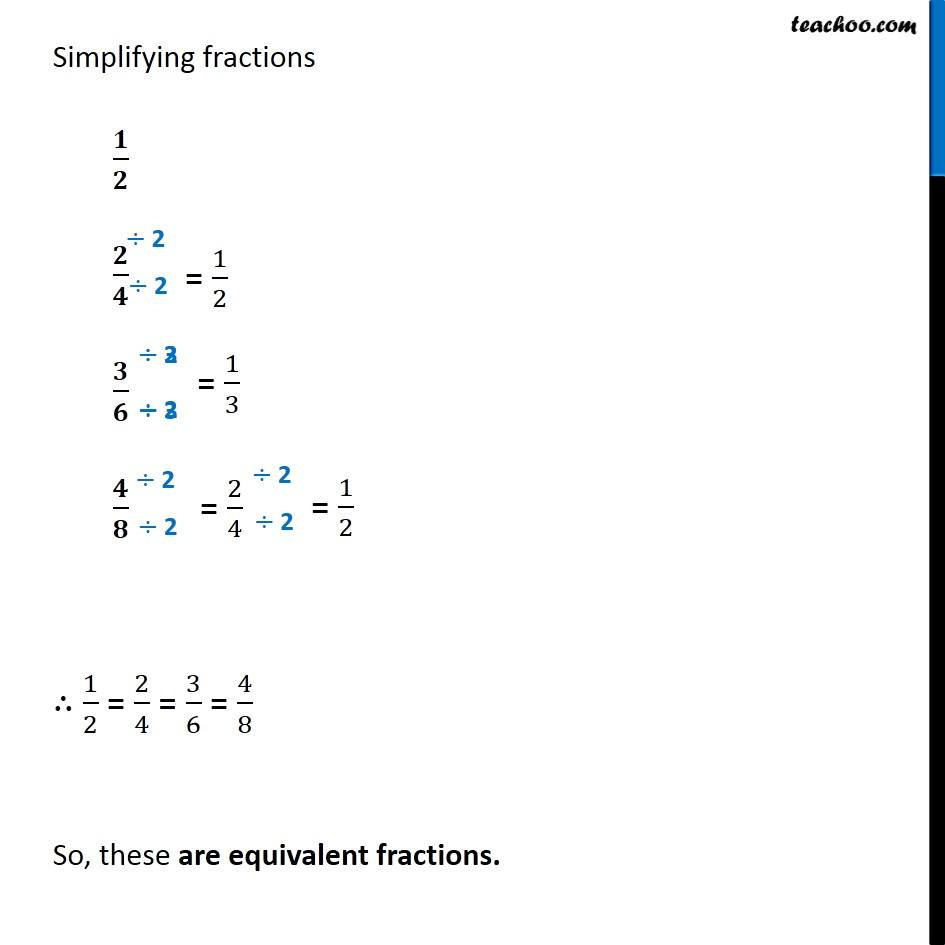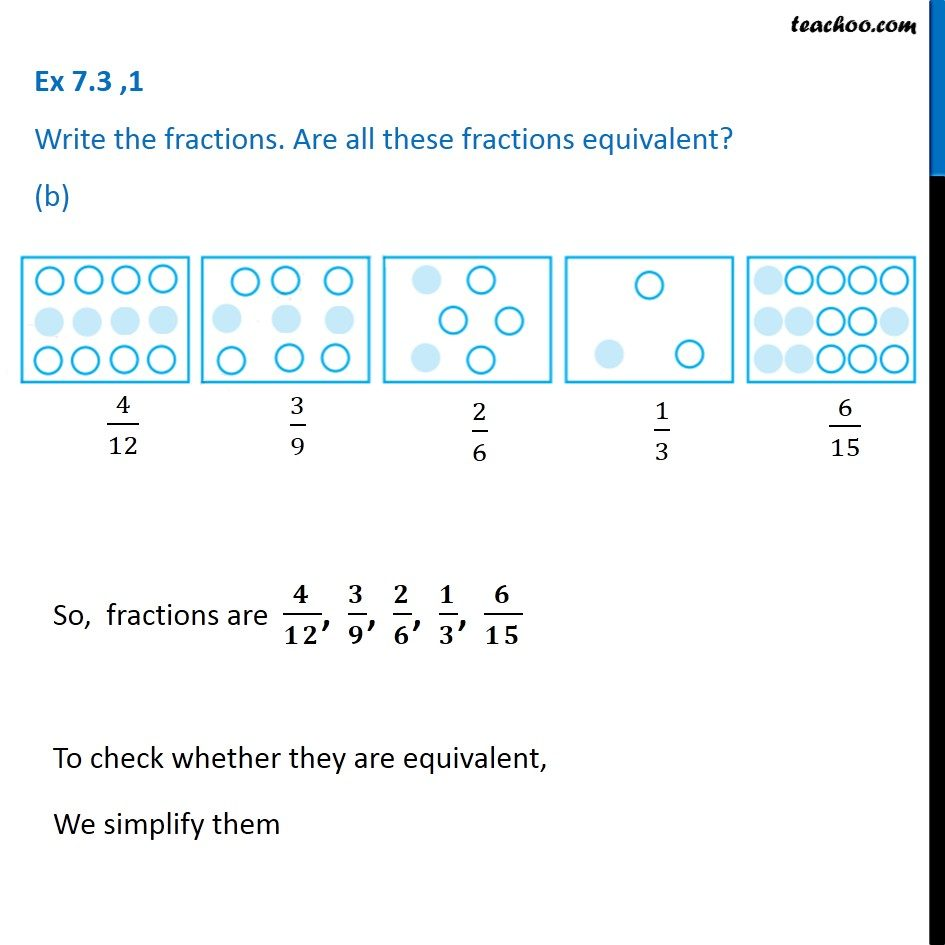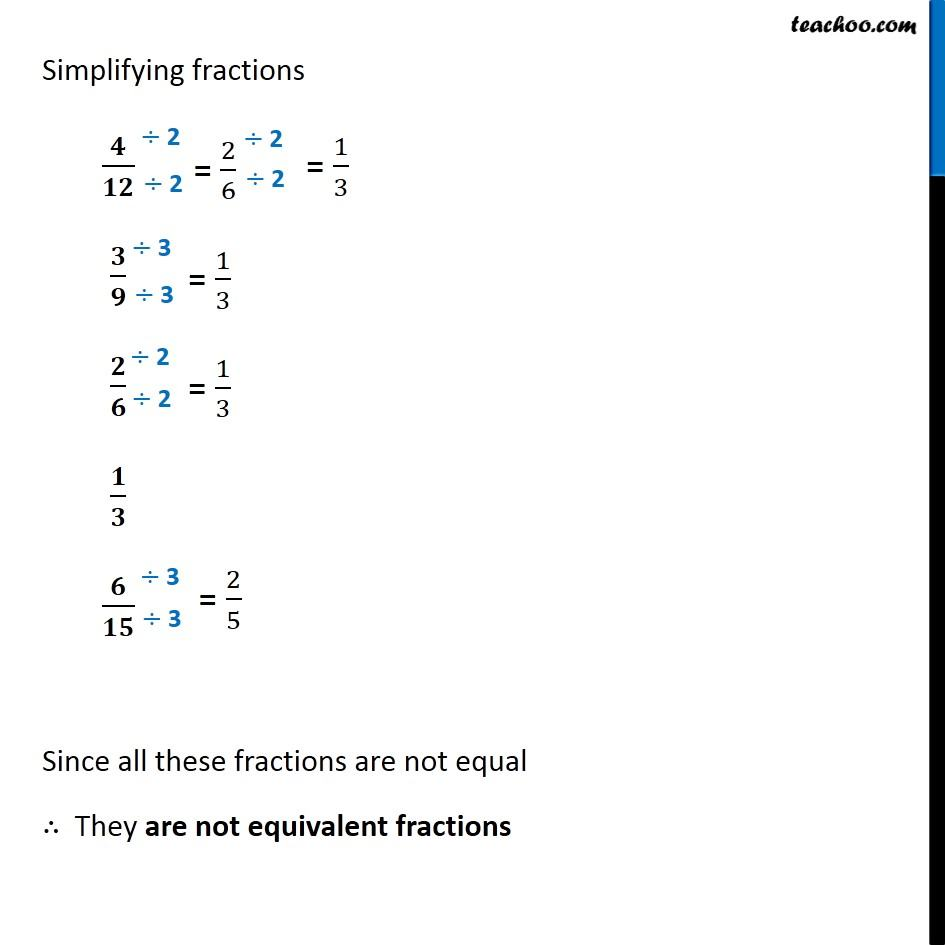Subscribe to our Youtube Channel - https://you.tube/teachoo

1. Chapter 7 Class 6 Fractions
2. Serial order wise
3. Ex 7.3

Transcript

Ex 7.3 ,1 Write the fractions. Are all these fractions equivalent? (a) So, fractions are 𝟏/𝟐, 𝟐/𝟒, 𝟑/𝟔, 𝟒/𝟖 To check whether they are equivalent, We simplify them Simplifying fractions 𝟏/𝟐 𝟐/𝟒 𝟑/𝟔 𝟒/𝟖 ∴ 1/2 = 2/4 = 3/6 = 4/8 So, these are equivalent fractions. Ex 7.3 ,1 Write the fractions. Are all these fractions equivalent? (b)So, fractions are 𝟒/𝟏𝟐, 𝟑/𝟗, 𝟐/𝟔, 𝟏/𝟑, 𝟔/𝟏𝟓 To check whether they are equivalent, We simplify them Simplifying fractions 𝟒/𝟏𝟐 𝟑/𝟗 𝟐/𝟔 𝟏/𝟑 𝟔/𝟏𝟓 Since all these fractions are not equal ∴ They are not equivalent fractions

Ex 7.3

Chapter 7 Class 6 Fractions
Serial order wise